Editor Ratings:
User Ratings:
[Total: 0 Average: 0]

Here is a list of 10 websites to calculate determinant of a Matrix. Matrices are a very crucial part of Mathematics course for students. However, solving determinant of any matrix is very easy for those which has an order of 2 0r 3 but the things get really tricky and annoying when the matrix is of higher order like 5 or 6.

So to help you with managing your time and solving the problems really fast, I have compiled the list of 10 websites to calculate determinant of a Matrix. Each of the websites have their own features and tools to enhance your user experience so let’s check them out one by one.

## 1. Matrix Reshish: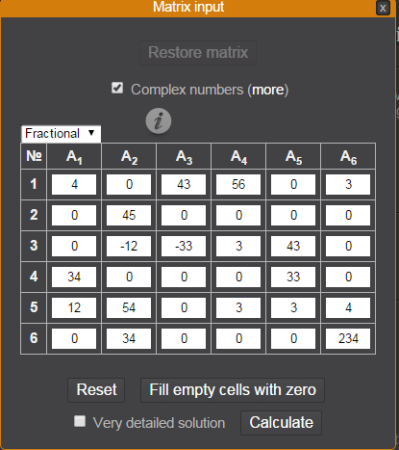Matrix Reshish is the first website to calculate determinant of a matrix. This website lets you choose the matrix of order up to 100 for finding the determinant. You can choose the preferred matrix order using the drop-down menu which has numbering from 2 to 100.

For getting the determinant, you have to enter the values in the given blank boxes. To decrease your time, you can simply enter all non-zero values in corresponding cell and then click on Fill empty cells with zero button to fill the zeros automatically. After this, you have to click on Calculate option to get the solution. The solution is shown to you on next page along with computation time. You can also opt for seeing the complete step by step solution.

Apart from all this, you can choose to enter values as Fractional or Decimal. The calculator also supports complex numbers for entering into matrix and getting the determinant.

## 2. QuickMath: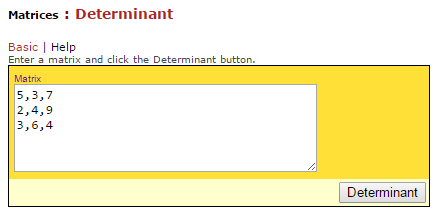As the name suggests, QuickMath is your destination for getting the determinant of any matrix quickly. This determinant calculator doesn’t have any separate cells for entering the values. You have to enter the values by separating two values using the comma.

After entering the values, click on Determinant option to get the answer. The website directly gives the answer along with options Click Here for Steps. You can click on it to get step by step solution.

## 3. Bluebit:Bluebit is also an easy to use determinant calculator and it supports matrix of order up to 32. There are no cells for entering numeral and you have to enter them like you do in simple notepad. You can choose the separator as comma, space, or tab. The website also lets you choose the decimal places to use for showing result. You can choose decimal places from 0 to 9 and 12, 15.

Clicking on Calculate option redirects you to the answer page where you will see the entered matrix along with solution. Apart from determinant, you can use this website for calculating Transpose, Trace, Rank, Inverse, Moore-Penrose Inverse, LU/LQ/QR/Cholesky/Singular Value Decomposition, and Eigenvalues/Eigenvectors.

## 4. Math Portal:The fourth determinant calculator in the list is Math Portal. It’s matrix calculator supports matrix of order up to 6 which you can choose using the slider. There are two portals for selecting the number of rows and columns respectively. It includes individual cells for entering values.

Below the cell, there are options to check Inverse, Trace, Transpose, Determinant, and Matrix Rank. You need to choose the solution you want and click on Compute to get the answer. When the answer is calculated, the website also gives you an option for saving it as PDF and for sharing the URL direct to solution page.

## 5. Math Is Fun: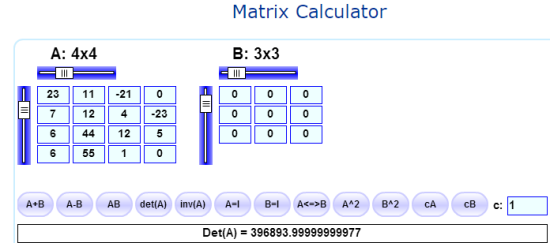Math Is Fun is the fifth determinant calculator website and just like its name, it makes it fun to calculate determinant. You can use this website to solve determinant of any matrix of order up to 10. Just like Math Portal, you have to use the sliders for setting the order of matrix.

The website supports a lot of actions which can be performed on matrix and all of them are given below the matrix. You have to click on option det(A) for getting the determinant of the matrix. You can see that there are two matrices in the screenshot. You have to enter the values in the matrix at right side only if you want to perform those actions which require two matrices like addition, subtraction, etc..

## 6. Number Empire: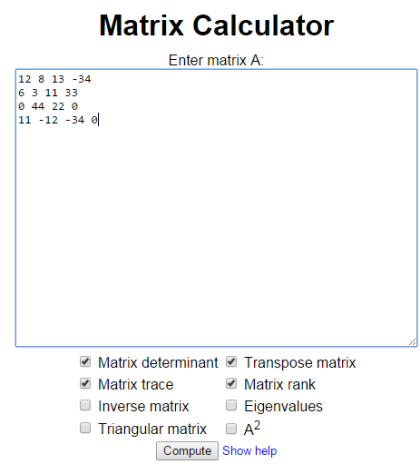Number Empire is one of the simplest determinant calculator in this list. It gives you a blank square in which you have to enter the values of matrix you want to solve. For separating two values from each other, you need to hit space while entering them. After entering values, choose Matrix Determinant and click on Compute option to get the answer.

Apart from calculating determinant, you can use it to calculate Trace, Transpose, Triangular Matrix, Inverse, Matrix Rank, Eigenvalues, and  A2.

## 7. Matrix Calculator: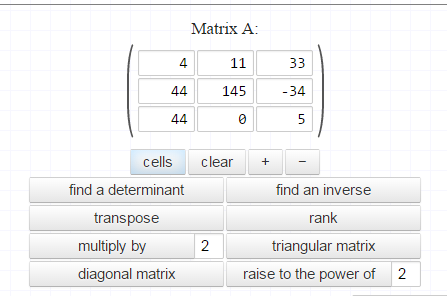The next determinant calculator website in the list is Matrix Calculator. While the website doesn’t mention the maximum order of matrix for solving the determinant but during my testing, I was able to solve till the order of 29 and I was still able to expand it to more. You can expand the matrix by clicking on + option and decrease it by clicking on – option.

When you have selected the order of matrix, enter values and click on Find A Determinant to get the answer. The website displays answer as well as gives option to check the step by step solution.

## 8. Solve My Math:The Solve My Math website also has an easy to use matrix calculator which is simple yet powerful. Like many of the calculators included in the list, you have to enter the values by using comma as the separator. When you have entered the values, choose the determinant from the options and click on Calculate to get the answer.

Apart from calculating determinant, you can use it to calculate Trace, Euclidian Norm, Normalized Determinant, Inverse, Transpose, and Scale Matrix.

## 9. Rechner Online: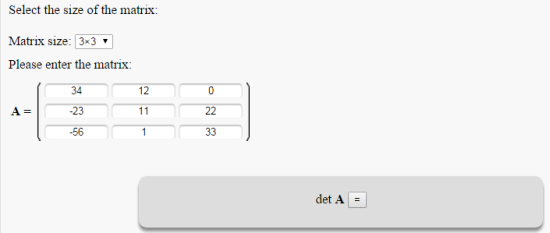The second last determinant calculator website is Rechner Online. It lets you choose the order of matrix as well as the number of decimal places for displaying the answer. You can choose the order of matrix up to 5 and number of decimal places up to 6. On selecting these, simply enter the values and click on Calculate to get the result.

## 10. OnlineMSchool:OnlineMSchool is the final determinant calculator website which is very simple to use and supports matrix of order up to 7. You can choose the matrix order using a simple drop down menu.  When you have entered the values, click on det A option to get solution. The website also displays step by step solution.

These were 10 websites to calculate determinant of matrix. Each of them have their own feature so try them to check out which one suits you best. If you face any problem or have any doubts, then feel free to get in touch with me via comments section below.

You may also like: 5 Free Websites to Solve Mathematics Problems Online.

 Editor Ratings: User Ratings:[Total: 0 Average: 0] Free/Paid: Free Tags: Matrix calculator